# Hardest colleges to get into in Iowa

Top 10 colleges in Iowa with the lowest acceptance rates
What are the hardest colleges to get into in Iowa? We've got you covered. We've compiled a national college database and have created a list of the hardest universities to get into in Iowa below. These are the hardest 4 year colleges to get into in Iowa, and sometimes some of the hardest colleges to get into in the US. You could even say these are the best colleges in Iowa. We also include each college's average SAT and ACT scores so that you can see where you're most competitive. Read on to find out more.

## Grinnell College acceptance rate

Grinnell College acceptance rate is 24.4%.The average SAT score for Grinnell College is 1460.

• SAT composite: 1460
• SAT math: 750

The average ACT score for Grinnell College is 32.

## Iowa Wesleyan College acceptance rate

Iowa Wesleyan College acceptance rate is 41.3%.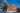The average SAT score for Iowa Wesleyan College is 1110.

• SAT composite: 1110
• SAT math: 560

The average ACT score for Iowa Wesleyan College is 21.

## Upper Iowa University acceptance rate

Upper Iowa University acceptance rate is 52.2%.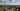The average SAT score for Upper Iowa University is 1050.

• SAT composite: 1050
• SAT math: 520

The average ACT score for Upper Iowa University is 21.

## Coe College acceptance rate

Coe College acceptance rate is 56.2%.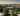The average SAT score for Coe College is 1200.

• SAT composite: 1200
• SAT math: 610

The average ACT score for Coe College is 25.

## Saint Ambrose University acceptance rate

Saint Ambrose University acceptance rate is 60.6%.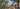The average SAT score for Saint Ambrose University is 1140.

• SAT composite: 1140
• SAT math: 570

The average ACT score for Saint Ambrose University is 23.

## Cornell College acceptance rate

Cornell College acceptance rate is 61%.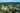The average SAT score for Cornell College is 1210.

• SAT composite: 1210
• SAT math: 600

The average ACT score for Cornell College is 27.

## Central College acceptance rate

Central College acceptance rate is 64.3%.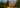The average SAT score for Central College is 1110.

• SAT composite: 1110
• SAT math: 530

The average ACT score for Central College is 24.

## Luther College acceptance rate

Luther College acceptance rate is 65.1%.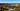The average SAT score for Luther College is 1165.

• SAT composite: 1165
• SAT math: 590

The average ACT score for Luther College is 26.

## Drake University acceptance rate

Drake University acceptance rate is 67.7%.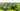The average SAT score for Drake University is 1248.

• SAT composite: 1248
• SAT math: 620

The average ACT score for Drake University is 27.

## Waldorf College acceptance rate

Waldorf College acceptance rate is 71.9%.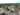The average SAT score for Waldorf College is 1030.

• SAT composite: 1030
• SAT math: 510